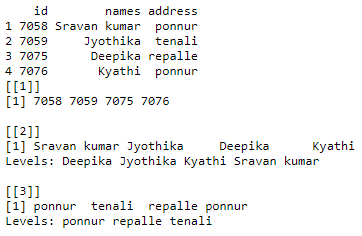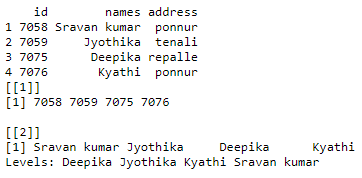# How to select multiple DataFrame columns by name in R ?

In this article, we will discuss how to select multiple columns from a DataFrame by name in R Programming Language. To get multiple columns we will use the list data structure. By using a list we can pass the dataframe columns separated with a comma. Then, we can get list by using list() function

Syntax:

list(dataframe_name\$column1,dataframe_name\$column2,.,dataframe_name\$column n)

Example1:

## R

 `# create a vector with student id ` `vector1=``c``(7058,7059,7075,7076) ` ` `  `# create a vector with student name ` `vector2=``c``(``"Sravan kumar"``,``"Jyothika"``, ` `          ``"Deepika"``,``"Kyathi"``) ` ` `  `# create a vector with student address ` `vector3=``c``(``"ponnur"``,``"tenali"``,``"repalle"``, ` `          ``"ponnur"``) ` ` `  `# pass these vectors to the dataframe ` `dataframe1=``data.frame``(id=vector1,names=vector2, ` `                      ``address=vector3) ` ` `  `print``(dataframe1) ` ` `  `# select multiple columns from the ` `# dataframe1 using list datastructure ` `a=``list``(dataframe1\$id,dataframe1\$names,dataframe1\$address) ` ` `  `print``(a) `

Output:Example 2:

## R

 `# create a vector with student id ` `vector1=``c``(7058,7059,7075,7076) ` ` `  `# create a vector with student name ` `vector2=``c``(``"Sravan kumar"``,``"Jyothika"``, ` `          ``"Deepika"``,``"Kyathi"``) ` ` `  `# create a vector with student address ` `vector3=``c``(``"ponnur"``,``"tenali"``,``"repalle"``,``"ponnur"``) ` ` `  `# pass these vectors to the dataframe ` `dataframe1=``data.frame``(id=vector1,names=vector2, ` `                      ``address=vector3) ` ` `  `print``(dataframe1) ` ` `  `# select multiple columns from the ` `# dataframe1 using list datastructure ` `a=``list``(dataframe1\$id,dataframe1\$names) ` ` `  `print``(a) `

Output:Whether you're preparing for your first job interview or aiming to upskill in this ever-evolving tech landscape, GeeksforGeeks Courses are your key to success. We provide top-quality content at affordable prices, all geared towards accelerating your growth in a time-bound manner. Join the millions we've already empowered, and we're here to do the same for you. Don't miss out - check it out now!

Previous
Next